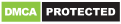# QANDA: Free Math Solutions

Genres
Version
-
Developer
-
Requires
-
Updated
-✨ Solve your doubts at QANDA! ✨

Have tricky homework or assignments? Studying for ACT/SAT exam? Need homework help right now?
QANDA is the one EASY solution you need.

✔ Need detailed solutions? From basic math to calculus, our smart calculator instantly gives you step-by-step solutions with a graph!
✔ Want to learn more? You can ask top-school tutors right away and get solutions within minutes.

Try out homework app chosen by over 10 million elementary, middle and high school students from 50+ countries!

▶ Main Features
Instant search
Struggling with homework? Just snap 📷 a photo and search solution in 5 seconds!
Learn with solved exercises and step-by-step explanations!
(And yes, this is 100% FREE!)

Web Search
No need to search things by yourself – all in QANDA now. 📚⚛️ 🧪📊🌎🎨
Find videos, step-by-step explanations, and more learning materials right away.

Smart calculator
Stuck with algebra, calculus or equation problems?
Just snap a math equation, and QANDA will give you multiple step-by-step instructions.
You can even get interactive graphs for equation problems too!

1:1 Q&A with tutors
Ask 1:1 questions directly to qualified top-school tutors right away!
In 1:1 Q&A, you can ask ALL subject questions including Physics and Chemistry! 👍

▶ Math Topic Covered in smart calculator
• Basic Math/Pre-Algebra: arithmetic, proportional, integers, fractions, decimal numbers, powers, roots, factors, complex numbers
• Algebra: linear equations/inequalities, quadratic equations/inequalities, general polynomial equations, systems of equations/inequalities, logarithms, functions, graphing, polynomials
• Trigonometry/Precalculus: identities, logarithmic functions, exponential functions, trigonometric functions
• Calculus: series, limits, derivatives, integrals
• Statistics: combinations, permutation, factorials

▶ Supported languages
English, Spanish, Korean, Japanese, Vietnamese, Indonesian, Thai

▶ Support & Feedback
We are always eager to know what you think and want to provide the best support for students’ educational endeavors.